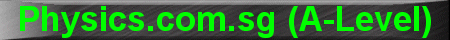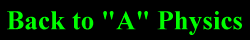(New tips are continually added to these pages.  Check back in a few months' time for more)

TOPIC 2:     Kinematics and Dynamics

Tip 1:

The 4 main equations in Kinematics are:

v = u + at
s = ut + Żat2
v2 = u2 + 2as
s =
[(u+v)/2] t

These equations should not be used in their raw form, as written above.  Instead, you should break them into x and y components, as follows:

vx = ux + axt            vy = uy + ayt
sx = uxt + Żaxt2          sy = uyt + Żayt2
vx2 = ux2 + 2axsx      vy2 = uy2 + 2aysy
sx =
[(ux+vx)/2] t      sy = [(uy+vy)/2] t

For example, in a projectile problem, where a canon ball is projected at a speed of 20 m s-1 at an angle of 30║ to the horizontal, it would be wrong to just let u = 20 m s-1 and start calculating.  Instead, you should use ux = 20 cos 30║ = 17 m s-1 and uy = 20 sin 30║ = 10 m s-1.

Tip 2:

Instead of just writing F = ma, it is better to write it as ňF = ma, since it is the resultant force that is equal to mass x acceleration.

The left hand side of the equation contains the resultant of all forces acting on the body.  Note that the weight of the body, mg, should be part of these forces (ie. on the left hand side of the equation), and not on the right hand side (a common mistake by students is to replace "ma" with "mg").

Tip 3:

When solving Dynamics problems, always:

1.    Draw the free-body-diagram, with all forces acting on the body.

2.    Indicate which direction to assign as the positive convention.

3.    Indicate the direction of acceleration and label it "a".